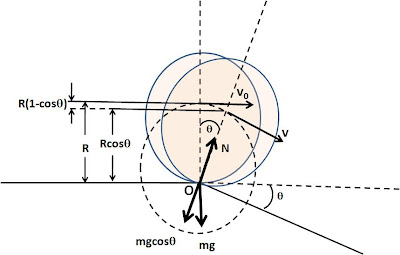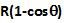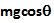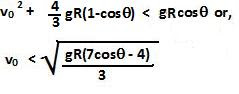## Friday, December 19, 2008

### Irodov Problem 1.264Initially the cylinder is rolling in the horizontal direction and fianlly the cylinder rolls in the direction of the slope. When the rolling cylinder reaches the corner point O, it has to change its direction of motion to roll down the slope. Thus, there is a transition time when the CG of the cylinder rotates (rolls) about point O until its direction of motion aligns with the final direction of motion (along the slope). By the time the cylinder's direction of motion has alinged with the direction of the slope, its CG will have fallen a height ofas shown in the figure. This fall in height of the CG results in a loss of gravitational potential energy which in turn results in an increase in the kinetic energy of the cylinder. In other words during this transition process of change of direction, the cylinder gains speed so that by the time its direction of motion has aligned with that of the slope (and the cylinder is about to start its journey down the slope) its speed v (in the direction of the slope) is greater than vo. The kinetic energy of the cylinder of mass m when rolling at a speed v is the sum of two parts, i) the translational kinetic energy ofand ii) a rotational kinetic energy of.

Since energy before and after the transition in the cylinder's direction of motion about point O, the energy should be conserved we have,

During the transition phase when the cylinder turns about the corner O to change its direction of motion, the CG moves along a circular path of radius R as shown in the figure. The cylinder will fly off of the surface if the centripetal acceleration due to this rotation about point O is so great that the force of gravity cannot provide for it. The higher the velocity of the cylinder as it rotates about point O, the greater is the danger of the cylinder's flying off. The maximum velocity the cyinder attains during the transition phase as its rotates about point O is given by v in (1). Thus, if it can sustain rotating about point O at speed v when it is about to start rolling down the slope, the cylinder will not fly off the corner point O. So let us consider, the point just before the end of the transition phase after which the cylinder will just about start rolling downwards.
There are two forces acting on the cylinder in the direction normal to the slope, i) the normal reaction from the surface N oriented along the perpendicular of the slope and ii) the component of the force of gravity. Since the cylinder's CG is still rotating about point O, at this point its acceleration if given by the centripetal acceleration directed towards O. So we have,If the cylinder flies off the surface then the normal reaction becomes 0 (since the cylinder is no longer in contact with the surface). So N=0 in (2) corresponds to the maximum v that can be sustained. So we have,

From (2) and (3) we have,